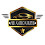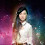### Skoda Car Logo Side Mirror Puzzle

This is a tricky math puzzle. You have to look closely at each image for solving the answer. Check for the correct answer below.

Explanation
Equation 1: 20 + 20 + 20 = 60   (So, Car = 20)
Equation 2: 3 x 3 x 3 = 27     (So, Emblem = 3)
Equation 3: (8 + 8) + (8 + 8) + 8 = 40    (So,  1 Side Mirror = 8)
Equation 4: (3 + 3) + [(20 - 3) - 8] x (20 - 16)    (Here, First Car do not have Emblem (-3) & do not have one side mirror (-8) ; Also the Second Car do not have both side mirrors (-16))
=> 6 + (9 x 4)
=> 6 + 36
=> 42

1.2.